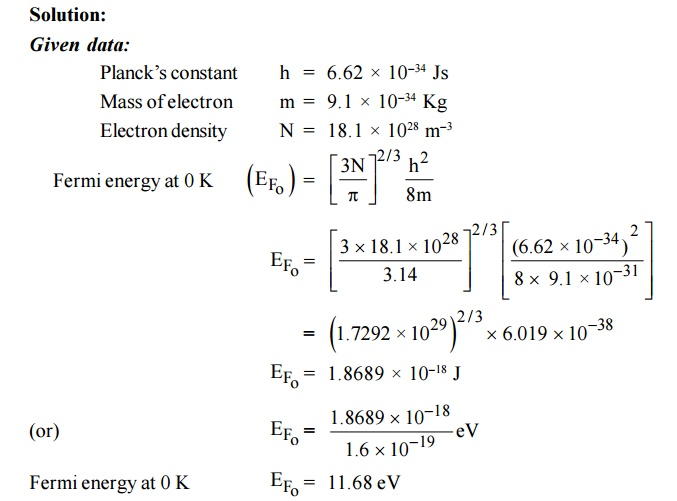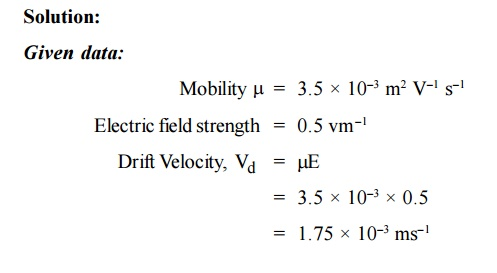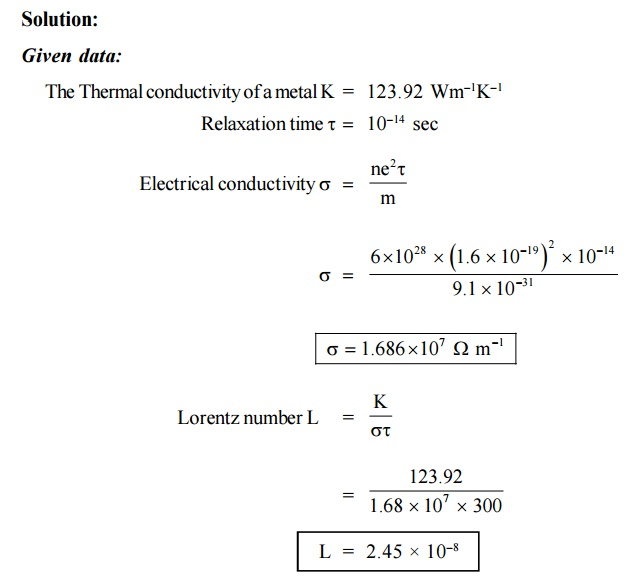Home | | Engineering Physics II | Solved Problems: Conducting Materials

# Solved Problems: Conducting Materials

Physics - Conducting Materials - Important Solved Problems: Conducting Materials

SOLVED PROBLEMS

1.The following datas are given for copper

Density = 8.92 × 103 kgm–3

Resistivity = 1.73 × 108    m

iii)Atomic weight = 63.5 kg

Calculate the mobility and the average time collision of electrons in copper obeying classical laws2.A uniform silver wire has a resistivity of 1.54×10–8 m at room temperature. For an electric field along the wire of 1 volt cm–1, compute the average drift velocity of electron assuming that there is 5.8 × 1028 conduction electrons /m3. Also calculate the mobility.3.The density of silver 10.5 × 103 kg–3 assuming that each silver atom provides one conduction electron. The conductivity of silver at 20°C is 6.8 × 107 –1 m–1. Calculate the density and mobility of electron in silver with atomic weight 107.9 × 10–3 kg m–2.4.Calculate the drift velocity of electrons in copper and current density in wire of diameter 0.16 cm which carries a steady current of 10 A. Given n = 8.46 × 1028 m–3.

Solution:

Given:

Diameter of the wire     d =    0.16 cm

Current flowing  =       10 A5. The resistivity of a piece of silver at room temperature 1.6 × 10–8 m. The effective number of conduction electrons is 0.9 per atom and the Fermi energy is 5.5 eV. Estimate the mean free path of the conduction electrons. Calculate the electronic relaxation time and the electronic drift velocity in a field of 100 Vm–1. The density of silver is 1.05 × 104 kgm–3 (m/m* = 1).6. A conducting rod contains 8.5 × 1028 electrons/m3. Calculate its resistivity at room temperature and also the mobility of electrons if the collision time for electron scattering is 2 × 10–14 sec.7. valuate the Fermi function for energy KBT above the Fermi energy.8. Free electron density of      aluminum is 18.1 × 1028 m–3. Calculate its  Calculate its  Fermi energy at 0 K. [Planck’s constant and mass of free electron are 6.62 × 10–34Js, and 9.1 × 10–34Kg]9.  The Fermi temperature of a metal is 24600 K. Calculate the Fermi velocity.10. Use the Fermi distribution function to obtain the value of F(E) for E – EF = 0.01 eV at 200K.

Solution:

Given data:11. Calculate the drift velocity of the free electrons (with a mobility of 3.5 × 10–3 m2 V–1 s–1) in copper for an electric field strength of 0.5 V m–1.12. Copper has electrical conductivity at 300 K as 6.40 × 107 m–1. Calculate the thermal conductivity of copper.(Lorentz number is 2.44 × 10–8 W k–2).13.The thermal and electrical conductivities of copper at 20°C are 380 Wm–1 K–1 and 5.67 × 107 –1 m–1 respectively. Calculate the Lorentz number.15. Calculate the Fermi energy of copper at 0 K if the concentration of electron is 8.5 × 1028 m–3.16. The mobility of electrons in copper 3×103m2V–1s–1 assuming e=1.6×10–19C and me=9.1×10–31 kg. Calculate the mean collision time.

Solution:17. The thermal conductivity of a metal is 123.92 Wm–1K–1. Find the electrical conductivity and Lorentz number when the metal posses relaxation time 10–14 sec at 300 K. (Density of electron = 6×1028 per m3)18. Calculate the drift velocity of the free electrons in copper for electric field strength of 2Vm–1. (Mobility of electrons µ = 3.5 × 10–3 m2V–1s–1).19. Find the velocity of copper wire whose cross-sectional area is 1 mm when the wire carries a current of 10 A. Assume that each copper atom contributes one election to the electron gas.ASSIGNMENT PROBLEMS

A Copper wire whose is 0.16 cm carries a steady current of 20 A. What is the current density of wire? Also calculate the drift velocity of the electrons in copper. (Ans : J = 9.952 A/m2 , and Vd = 7.35 x 10- 4 ms-1)

The thermal and electrical conductivities of Cu at 20°C are 390 Wm 1 K 1and 5.87 × 10–7 –1 m–1respectively. Calculate the Lorentz number. (Ans : 2.267 × 10–8 W          K–1)

Calculate the electrical and thermal conductivities of a metal rod with relaxation time 10 14 second at 300K. Also calculate the Lorentz number.

(Density of electron = 6 × 1028 m–3)(Ans :  = 1.6879 × 10–7    –1       m–1 ,  K = 123.927 Wm–1  K –1 , = 2.4474 × 10–18 W  K–2)

Calculate the drift velocity and mean free path of copper when it carries a steady current of 10 amperes and whose radius is 0.08 cm. Assume that the mean thermal velocity 1.6 × 106 m/s and the resistivity of copper 2 × 10–8 m.

(Ans : (i) 36.6 x 10-5m/s (ii) 3.94 x 108m)

The resistivity of aluminum at room temperature is 2 × 10–8 m.Calculate i) The drift velocity ii) mean free path on the basis of classical free electron theory.

(Ans : (i) 0.396 ms-1; (ii) 2.65nm)

6.  Using the Fermi function, evaluate the temperature at which there is 1% probability in a solid will have an energy 0.5 eV above EF of 5 eV. (Ans : 1260 K)

Study Material, Lecturing Notes, Assignment, Reference, Wiki description explanation, brief detail
Physics : Conducting Materials : Solved Problems: Conducting Materials |